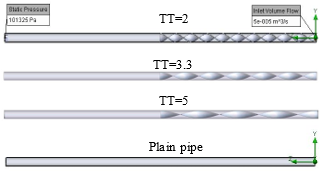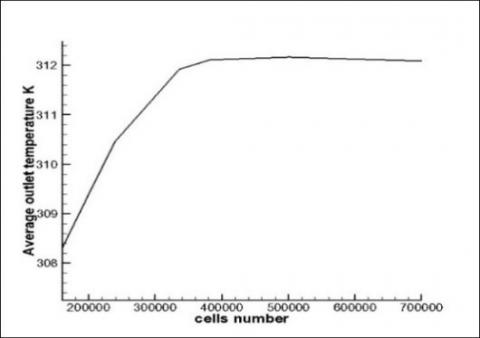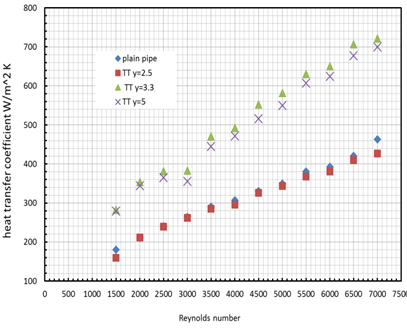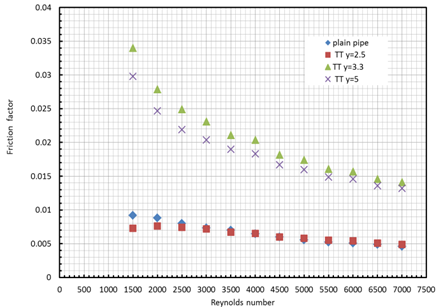# Effect of Twisted Tape Ratio on the Solar Generator Half-length Pipe

Effect of Twisted Tape Ratio on the Solar Generator Half-length Pipe

Corresponding Author Email:
Dhafer.A.Hamzah@qu.edu.iq
Page:
407-412
|
DOI:
https://doi.org/10.18280/ijht.370205
9 December 2018
|
Accepted:
2 April 2019
|
Published:
30 June 2019
| Citation

OPEN ACCESS

Abstract:

The effect of twisted tape ratio has been studied numerically to promoting a swirl effects inside a long half-length pipe. A constant wall temperature demonstrates solar collector at average temperature conditions for the receiver pipe. The flow simulation adjusted at different range of Reynolds number 1500-7000 and varied twisted tape ratio y=2, 3.3, and 5. The results showed that; when the twisted tape pipe increases (for example at 3.4), the average outlet temperature, heat transfer coefficient, Nusselt number, friction factor, and power losses increases to 64, 65, 28, 14.1, 31 % respectively compared with plain pipe, also the average outlet fluid temperature is maximum from 305 K-322 K at low Reynolds number 1500 for all cases and then will be decreasing with increasing Reynolds number .The results presented in contours of temperature distribution and flow trajectory by axial velocity were accomplished to exam the low Reynolds number in solar applications. It showed that, when the swirl geometry used, which would be effect on the axial velocity for the flow by 10 % more than the cases without twist tape.

Keywords:

twist tape, thermo- hydraulic, friction factor, CFD, swirl flow

1. Introduction

Derangement of earth environment caused by emission of fossil fuel stimulate the researchers for finding out new techniques to increase the available work and decreasing destructing energy. Nanofluid, twisted tape inserte in pipe, and extended surface are some wayes used to increase the heat transfer and the thermal efficiency of the system.

In general, many experimental articles concern in the field of enhancement thermal performance and evaluate hydraulic characteristics. Kumar and Prasad  studied the effect of insert twisted tape in solar water heater with pitch ratio (3-12) to promote swirl generator for various flow rate. They found that the range of heat transfer increases by 18-70 % and the pressure drop increases by 87-132 % compared with plain pipe. Another study made by Promvonge and Eiamsa-ard  using conical ring as tabulator supported with twisted tape as vortex generator at the core in a tube impose to axially and circumferentially heat flux. Two different twisted tape ratio (3.75 and 7.5) have been used. The airflow thermal performance of conical coil (4 %-8 %) greater than that with untwisted tape conical ring. Jaisankar et al.  considered the destructing of the sub layer in the solar collector for Reynolds number (3000-23000) and twist ratio (3, 4, 5 and 6). The results indicated that; the heat transfer performance and pressure drop increase in case of twist ratio 3 rather than plain pipe collector. The fluid flow in peripherally cut twisted tape tube expose to uniform heat flux have been investigated experimentally by Seemawute and Eiamsa-ard . Naphon and Suchana  concerned on the high performance and friction factor for brush twisted wire of the conjugate heat transfer heat exchanger working with different hot inlet temperature. The twisted wire provided with copper brushes at the core with different densities, also the insert wire were a long full length and regular region. High Reynolds number considered between 6000-20000 and the hot and cold temperature were 40-50o and 15-20o respectively. Murugesan et al.  worked on effect different depth and width ratio of V- cut twisted tape on the thermal and hydraulic performance. Nusselt number and friction factor increase inversely with twist and width ratios at low values of depth ratios. A Computational Fluid Dynamic (CFD) technique have been used to simulate twisted tape inside circular tube with different ratio and locations by Salman et al. . There results shown, both of heat transfer and hydraulic characteristics increases when the twist ratio decreases. Another simulation done by Yadav and Padalkar  to prepare boundary conditions of heat exposure regions and configurations to generate swirl effect with range of twisted ratio. The obtained results pointed out that; the heat transfer coefficient as well as pressure drop in the plan tube with the FLTT more than the normal tube cases and it were 29-86% and 203-623% respectively. The effects of different shapes of inserted tape stared, coiled and typical with REN k-ϵ model for turbulent flow investigated numerically by Goodarzi et al. . The results shown that, a high thermal performance and friction factor occurs with all types above especially starred types. Piriyarungrod et al.  examined the effect of the different tapered angle (0o, 0.3o, 0.6o and 0.9o) and twist ratio (3.5, 4, and 4.5) on the heat transfer and pressure drop. The flow regime for all cases was turbulent flow. Furthermore, the Reynolds number selected in the study between (6000 and 20000). The results showed, the higher thermal performance becomes when the taper angle equal to 0.9o and the twist ratio equal to 3.5. Kaliakatsos et al.  analyzed the mixing effect of high viscous fluid and water in industrial heat exchanger by using twisted tape with different Reynolds number. Their results show that; there are five effects of compositions led to increased convective heat transfer coefficient. Promvonge and Eiamsa-ard  studies the three dimensional CFD modeling for various shapes of inserted tape inside heat exchanger. It has been used three different twisted tapes (plain, jagged V-cut, and V-cut) in addition to using two different twisted ratio equal to (2 and 4). The CFD study found that; when using the jagged V- cut twisted tape, the high thermal performance factor take place comparing with other cases. A Numerical investigation made by Mashoofi et al.  to study the effects of axial perforated twisted tapes in double tube heat exchanger. The result shows that; the effectiveness and the friction factor for the heat exchanger with PTTs decrease (from 2.17 to 10.5 %) and (from 8.5 to 44.2 %) respectively, comparing with the heat exchanger with STT. Singh Suri et al. , their experimental work was on the tube of heat exchanger with square hole a long wing tape. The study concerned on the effect of wing depth, perforate depth and twist ratio. They reported Nusselt number and friction factor were increased 6.96 and 8.34 times respectively in range of Reynolds number between (5000 and 27000). Recently Sarma et al.  studied the thermal performance of heat exchanger tubes; on the other hand, Chaware and Sewatkar  have studied the effects of the velocity on the heat transfer for flow through pipe with twisted tape insert-turbulent flow. Recently, great studies have been done with/without nanofluid flow in a tube with effects of twisted tape [16-17].

From the above survey extreme Reynolds number were considered while the solar receiver/collector working at low range of Reynolds number to give enough time to reach the operating temperature, for example (solar refrigerator).

The present work depends on classic case of insert twist tape at low range of Reynolds number then transition and turbulent flow. The Main purpose of this study is to show the near values of twist ratio, which gives the same thermal and hydraulic behavior at same Reynolds number.

2. Problem and Boundary Conditions Description

Three-dimension computational fluid dynamics (CFD) have been used to consider the effects of twisted tape ratio inside a long half-length pipe. The pipe length selected to be equal to 2 m while, the pipe diameter chosen to be 5 cm. A twisted tape inserted inside the second half-length of the pipe as shown in Figure 1. In this study, the wall pipe assumed to be at constant temperature (333 K), and the inlet water temperature assumed to be constant at (293 K) for all cases. The diameter to the pitch ratio (TT) is 2.5, 3.3, and 5. Reynolds number takes from (1500 to 7000) for each twist ratio.

## 1.pngFigure 1. Plain pipe and the pipe with twist tape ratio

3. Mathematical Model

In this study, the three-dimensional governing equations including; conservation equation of mass, momentum and energy equations of the fluid flow in the straight and curved tubes have been solved numerically by using finite element methods.

The three-dimensional equations can be written as follows:

$\frac{\partial \rho}{\partial t}+\frac{\partial}{\partial x_{i}}\left(\rho u_{i}\right)=0$    (1)

$\frac{\partial \rho u_{i}}{\partial t}+\frac{\partial}{\partial x_{j}}\left(\rho u_{i} u_{j}\right)+\frac{\partial p}{\partial x_{i}}=\frac{\partial}{\partial x_{j}}\left(\tau_{i j}+\tau_{i j}^{R}\right)+S_{i}$   (2)

$\frac{\partial \rho H}{\partial t}+\frac{\partial \rho u_{i} H}{\partial x_{i}}=\frac{\partial}{\partial x i}\left(u_{j}\left(\tau_{i j}+\tau_{i j}^{R}\right)+q_{i}\right)+\frac{\partial p}{\partial t}-\tau_{i j}^{R} \frac{\partial u_{i}}{\partial x_{j}}+\rho \varepsilon+S_{i} u_{i}+Q_{H}$    (3)

The mean velocity and Reynolds number for circular pipe can be calculated as follow:

$u_{m}=\frac{4 m}{\pi \rho D_{i}}$  (4)

$R e=\frac{\rho u_{m} D_{i}}{\mu}$     (5)

$f=\frac{64}{R e}$   for laminar and plain pipe       (6)

$f=\frac{\Delta p}{(L / d) \rho u^{2} / 2}$  for turbulence and twist tape pipe   (7)

Nusselt mumber $=\frac{\left(\frac{f}{8}\right)(\operatorname{Re}-1000) \operatorname{Pr}}{1+12.7\left(\frac{f}{8}\right)^{0.5}\left(\operatorname{Pr}^{\frac{2}{3}}-1\right)}$    (8)

## 2.jpgFigure 2. Grid sensitivity check

CFD SolidWorks software has been chosen in this study to solve the governing equations along with the boundary conditions. SolidWorks fluid flow simulation is a finite element analysis package using for various physics and engineering applications. In this study, mesh generation on long half-length pipe with/without twisted tape. The grid sensitivity check is shown in Figure 2 for determination whether the scheme of mesh is sufficient. Many grid sensitivity tests to ensure that the results are grid independent and 3800,000 cells number was selected for all the computations done in the present work.

4. Results and Discussion

The thermal and hydraulic comparisons presented in this section to evaluating the performance to each case of the pipe with and without insert twisted tape. The harvest of different surface parameters from software programme will be insert in continuity, momentum and energy equations to have the objective variables.

4.1 Thermal behavior

Figure 3 presented the thermal behavior of each case including; the average outlet temperature, the heat transfer coefficient and the Nusselt number versus Reynolds number for different twisted tape ratio. The average outlet temperature is an important factor to evaluate how much gained heat. In Figure 3a, the average outlet fluid temperature is maximum at low Reynolds number (Ra=1500) for all cases. It always decreases when Reynolds number increases, which happen due to low mass flow rate, which will give enough time to heat transfer.

It can be seen that the maximum outlet water temperature occurs with the cases of twist ratio equal to 3.3 in spite of case twist ratio 5 have long pitch. That has happened; due to the flowing path is long as well as the demonstrating drag, which will late the flow of fluid for taking more heat from the source. The results conclude that pipe with twist tape have a good thermal performance comparing with plain pipe (more than 90 %). In Figure 3b, the heat transfer coefficient increases with increases of Reynolds number for all cases, because of increasing the turbulent generator and mixing fluid flow due to swirl motion. To complete the picture of thermal characteristics, Nusselt number reports, which is, dominated convection/conduction near the wall as shown in Figure 3c. It can be seen that, the Nusselt number increase when Reynolds number increase, furthermore it is increasing with increasing of twist tape until (TT=3.3) and then it decreases.

## 3a.pnga

## 3b.pngb

## 3c.pngc

Figure 3. Average of (a) outlet temperature, (b) heat transfer coefficient and (c) Nusselt number versus Reynolds number for plain pipe and pipe with different twist tape ratio

4.2 Hydraulic behavior

Thermal efficiency must be increasing without a burden on the hydraulic performance. In this section, an evaluation to the friction factor and turbulent intensity will be discussed.

More time of residence to the flow will prolong heat transfer time for thermal behavior. This shown in the Figure 4a, which indicates the friction factor versus Reynolds number.

The results showed, friction factor decreasing with Reynolds number increasing for all cases, due to the swirl and mixing in the boundary layer phenomena. The highest friction factor is equal to 0.034, for the case of twist ratio 3.3 at the low Reynolds number. Figure 4b represented the kinetic energy dissipation as turbulent intensity behavior (ratio of fluctuating velocity to the average velocity) versus Reynolds number. Turbulent intensity decreases when Reynolds number increase, i.e. the flow in the pipe with twist tape is less kinetic energy dissipation than plain pipe. This mean the flow in the pipe with twist tape have less values of fluctuating velocity and maintain constant flow, in other words more turbulent intensity led to increase in flow path. A pumping power loss is important parameters for overall performance as Nusselt number. The twist tape must not work as a very obstructive to the flow.

A balance must be made between drag and promote the thermal performance. Figure 4c, represent the power losses due to insert twist tape and plain pipe. Twist ratio cases of 3.3 and 5 have highest power loss equal to 0.011W at Reynolds number 7000.

Also an evident to the same power losses of Twist ratio y=2 and plain pipe is almost same at Reynolds 1500-7000.

## 4a.pnga

## 4b.pngb

## 4c.pngc

Figure 4. (a) Friction factor, (b) Turbulent intensity, and (c) Pumping power losses versus Reynolds number for plain pipe and pipe with different twist tape ratio

4.3 Visualization contours

The visualization contours give deep vision in the behavior of the parameters in the mini different region. The results discussed in fluid temperature and flow trajectory in z-velocity contours for plain pipe and twist tape pipe (TT, y=3.3) at laminar flow for (Ra=1500) and turbulent flow for (Ra=7000).

Figure 5 shows the temperature distribution for heat transfer between wall and fluid flow. The results indicate when Reynolds number increase, the temperature gradient decrease due to high flow rate for both cases. The effect of twist tape is shown on the distribution temperature even on the first half of pipe. It can be seen the variation temperature on all the pipe length especially for low Reynolds number. In twist tape region, the heat transfer increases due to increasing of swirl flow. The white tiny region a long half pipe indicates to the solid twist material and no fluid in this cut plot region. The range of maximum temperature due to twist tape are 330 K for laminar flow and 312 K for turbulent.

## 5.pngFigure 5. Temperature distribution comparison between plain pipe and the pipe with twist tape

## 6.pngFigure 6. Flow trajectory colored by z-velocity comparison between plain pipe and the twist tape pipe

Figure 6 shows the swirl motion demonstration due to twist tape for twist ratio TT=3.3 with z- velocity, which is increasing with Reynolds number increasing. The range of velocity start from 0.035 m/s to 0.141 m/s for twisted tape pipe, while the range for z- velocity start from 0.09 m/s to 0.154m/s for plain pipe, since the drag by the twist tape which increase the friction factor.

5. Conclusions

Classic conclusions are known such as heat transfer increase with twist ratio increase as well as friction factor, therefore, this study tries to conclude new hidden points.

1. The effect of twist tape is clear in low Reynolds number, i.e. thermal performance increase with decrease twist tape. This is coincident with requirements conditions of solar collector.
2. The high twist ratio means more heat transfer for same bulk volume of twist material
3. Some near twist ratio gives the same thermal and hydraulic behavior, so the difference in twist values must be more than 1.
4. For passive solar system, friction factor considers secondary parameter comparing with heat transfer coefficient, due to there is no pumping power.
5. The swirl geometry effected on the axial velocity for the flow by 10% more than the cases without twist tape.

Turbulent intensity considers a helpful parameter to swirl motion analysis toward increase heat transfer.

Nomenclature
 D Diameter f Friction factor h Heat transfer coefficient k Thermal conductivity Greek symbols μ Dynamic viscosity ρ Density
References

 Kumar, A., Prasad, B.N. (2000). Investigation of twisted tape inserted solar water heaters—heat transfer, friction factor and thermal performance results. Renewable Energy, 19(3): 379-398 https://doi.org/10.1016/S0960-1481(99)00061-0

 Promvonge, P., Eiamsa-ard, S. (2007). Heat transfer behaviors in a tube with combined conical-ring and twisted-tape insert. International Communications in Heat and Mass Transfer, 34(7): 849-859. https://doi.org/10.1016/j.icheatmasstransfer.2007.03.019

 Jaisankar, S., Radhakrishnan, T.K., Sheeba, K.N. (2009). Experimental studies on heat transfer and friction factor characteristics of forced circulation solar water heater system fitted with helical twisted tapes. Solar Energy, 83(11): 1943-1952. https://doi.org/10.1016/j.solener.2009.07.006

 Seemawute, P., Eiamsa-ard, S. (2010). Thermohydraulics of turbulent flow through a round tube by a peripherally-cut twisted tape with an alternate axis. International Communications in Heat and Mass Transfer, 37(6): 652-659. https://doi.org/10.1016/j.icheatmasstransfer.2010.03.005

 Naphon, P., Suchana, T. (2011). Heat transfer enhancement and pressure drop of the horizontal concentric tube with twisted wires brush inserts. International Communications in Heat and Mass Transfer, 38(2): 236-241. https://doi.org/10.1016/j.icheatmasstransfer.2010.11.018

 Murugesan, P., Mayilsamy, K., Suresh, S., Srinivasan, P.S.S. (2011). Heat transfer and pressure drop characteristics in a circular tube fitted with and without V-cut twisted tape insert. International Communications in Heat and Mass Transfer, 38(3): 329-334. https://doi.org/10.1016/j.icheatmasstransfer.2010.11.010

 Salman, S.D., Kadhum, A.A.H., Takriff, M.S., Mohamad, A.B. (2014). CFD simulation of heat transfer augmentation in a circular tube fitted with alternative axis twisted tape in laminar flow under a constant heat flux. Heat Transfer—Asian Research, 43(4): 384-396. https://doi.org/10.1002/htj.21089

 Yadav, R.J., Padalkar, A.S. (2012). CFD analysis for heat transfer enhancement inside a circular tube with half-length upstream and half-length downstream twisted tape. Journal of Thermodynamics, 2012: 1-12. http://dx.doi.org/10.1155/2012/580593

 Goodarzi, K., Goudarzi, S.Y., Zendehbudi, G. (2014). Investigation of the effect of using tube inserts for the intensification of heat transfer. Thermal Engineering, 62(1): 68-75. https://doi.org/10.1134/S004060151501005X

 Piriyarungrod, N., Eiamsa-ard, S., Thianpong, C., Pimsarn, M., Nanan, K. (2015). Heat transfer enhancement by tapered twisted tape inserts. Chemical Engineering and Processing: Process Intensification, 96: 62-71. https://doi.org/10.1016/j.cep.2015.08.002

 Kaliakatsos, D., Cucumo, M., Ferraro, V., Mele, M., Galloro, A., Accorinti, F. (2016). CFD analysis of a pipe equipped with twisted tape. International Journal of Heat and Technology, 34(2): 172-180. https://doi.org/10.18280/ijht.340203

 Mashoofi, N., Pourahmad, S., Pesteei, S.M. (2017). Study the effect of axially perforated twisted tapes on the thermal performance enhancement factor of a double tube heat exchanger. Case Studies in Thermal Engineering, 10: 161-168. https://doi.org/10.1016/j.csite.2017.06.001

 Singh Suri, A.R., Kumar, A., Maithani, R. (2017). Effect of square wings in multiple square perforated twisted tapes on fluid flow and heat transfer of heat exchanger tube. Case Studies in Thermal Engineering, 10: 28-43. https://doi.org/10.1016/j.csite.2017.03.002

 Sarma, P., Konijeti, R., Subramanyam, T., Prasad, L., Korada, V., Srinivas, V., Vedula, D., Prasad, V. (2017). Fouling and its effect on the thermal performance of heat exchanger tubes. International Journal of Heat and Technology, 35(3): 509-519. https://doi.org/10.18280/ijht.350307

 Chaware, P., Sewatkar, C. (2017). Effects of tangential and radial velocity on the heat transfer for flow through pipe with twisted tape insert-turbulent flow. International Journal of Heat and Technology, 35(4): 811-820. https://doi.org/10.18280/ijht.350417

 Tusar, M., Noman, A., Islam, M., Yarlagadda, P., Salam, B. (2019). CFD study of heat transfer enhancement and fluid flow characteristics of turbulent flow through tube with twisted tape inserts. Energy Procedia, 160: 715-722. https://doi.org/10.1016/j.egypro.2019.02.190

 Habeeb, L., Saleh, F., Maajel, B. (2019). CFD modeling of laminar flow and heat transfer utilizing Al2O3/water nanofluid in a finned-tube with twisted tape. FME Transactions, 47(1): 89-100. https://doi.org/10.5937/fmet1901089H